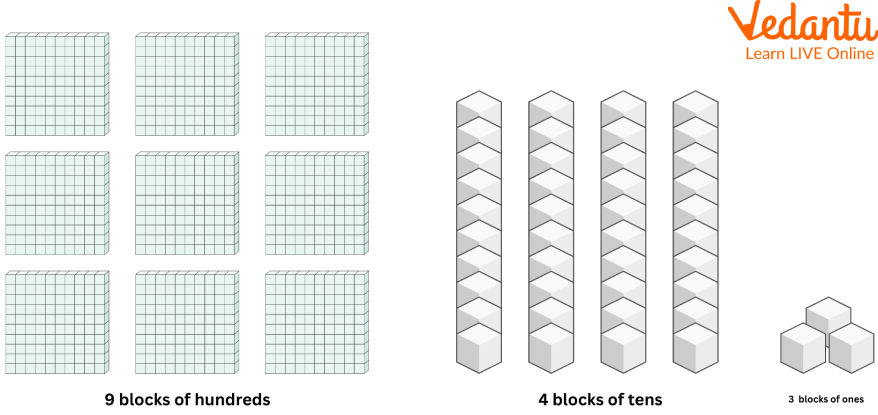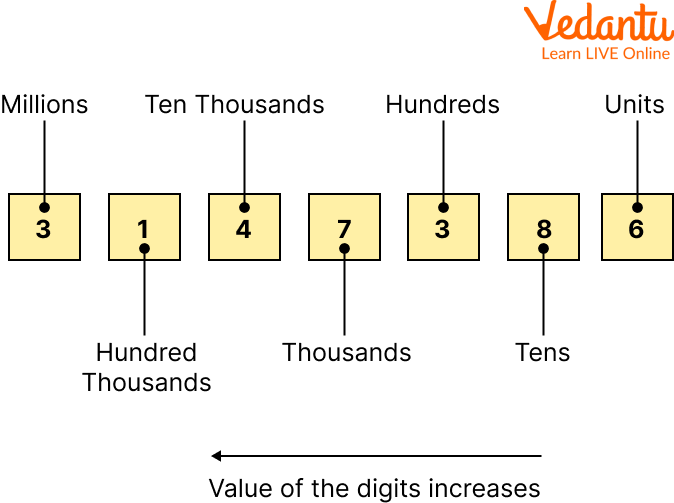Courses
Courses for Kids
Free study material
Free LIVE classes
More

# Add Using the Expanded FormLIVE
Join Vedantu’s FREE Mastercalss

## A Sneak Peek over the Expanded Form

Ever wondered how a huge number can be broken down into small parts? This concept in mathematics is called the Expanded form. If you are unaware of this, you don’t need to worry. Because in this article, we will discuss everything about the expanded form and how we can add using expanded form. We will learn how to express the digits using addition in expanded form and expanded form sums from scratch, along with solved examples. Moreover, we will focus on how to add using the expanded form.

## What is an Expanded Form?

Expanded form is a way to write a number by adding the value of its digits. Use a place value chart to get a clear idea about the value of a number's digits.

In an expanded form, a number is broken according to its place value and expanded to show the value of each digit. When we add using the expanded form, it makes it easy to understand, and by solving expanded form sums, we can develop a better understanding of it. For example, 943 can be written in expanded form, as given below.

The expanded form of 943 is given by 9 hundred 4 tens 3 ones.

expanded form: 900 + 40 + 3.An Example of an Expanded Form

## Place Value

Each number has a place value. The value of that digit is determined according to its position in the number. As we move from left to right, the value of a digit in a number increases. The place value of the digits on the left is lesser than those on the right.

By using the position of the number, the value for each number is computed. On starting from right to left, we can understand the notations used in the place value using an example.Place Value of a Number

Thus, the expanded form of the number 254 is 200 + 50 + 4.

## How to Find an Expanded Form?

Steps to write a number in the expanded form are given below.

Ones: $1 \times 4=4$

Tens: $7 \times 10=70$

Hundred: $3 \times 100=300$

Thousand: $8 \times 1000=8000$

$8000+300+70+4=8374$

• Write the number in its most basic form.

• Use the place value table to determine its place values.

• Let the place value of the number be Multiplied by the number.

• The number is multiplied by the number’s place value.

• Display it as a digit place value.

• Each digit should be represented as the product of the digit and its place value.

## Expanded Form with Decimals

The extended form of a number with a decimal point or fraction is written with a base 10 denominator represented by a power of 10. For example, the number 3.482 in the expanded form is written as:

3.482 = 3 + 0.4 + 0.08 + 0.002.

## How to Write in Expanded Form?

Suppose we want to expand 1.234. At first, we have one place, i.e. 1. Then, we have the first decimal place, the tenths. We take 2 and multiply it by a fraction $\dfrac{1}{10}$

$1+\left(2 \times \dfrac{1}{10}\right)$

Then, we have the hundredth place. Now move to a higher multiple of 10 for the denominator. In simple words, add another 0.

$1+\left(2 \times \dfrac{1}{10}\right)+\left(3 \times \dfrac{1}{100}\right)$

Finally, we have the thousandth place. Add another 0 in the denominator.

$1+\left(2 \times \dfrac{1}{10}\right)+\left(3 \times \dfrac{1}{100}\right)+\left(4 \times \dfrac{1}{1000}\right)$

Hence, the expanded form of $1.234$ is $1+0.2+0.03+0.004$.

With the help of an example, we will learn about addition in expanded form.

32= 30 + 2 (An expanded form of the number)

25= 20 + 5

Now, 32+25=30+2+20+5

= 50 + 7

= 57

The numbers are written in expanded form as 32 = 30 + 2 and 25 = 20 + 5.

Now digits of one’s place are added, i.e., 2 + 5 = 7.

Again numbers of ten’s place are added, i.e., 30 + 20 = 50

Total sum = 50 + 7 = 57

Thus the required total sum is 57.

## Solved Expanded Form Sums

1. Add 361 and 527 by arranging the numbers in expanded form.

361 = 300 + 60 +1

527 = 500 + 20 +7

Sum = 800 + 80 + 8

= 888

Thus the required sum is 888.

2. Add using the expanded form: 231, 402, and 355

Adding ones = 1 + 2 + 5 = 8

Adding tens = 3 + 0 + 5 = 8

Adding hundred = 2 + 4 + 3 = 9

Therefore, the addition sum is 988.

1. 31 + 42 =

Ans. 73

Q2. 43 + 44 =

Ans. 87

Q3. 23 + 25 =

Ans. 48

## Summary

In this article, we learned that the expanded form is nothing more than a technique for rewriting a number by including the values of the digits. With the help of some solved expanded form sums, we tried to understand how numbers are arranged in the expanded form before adding.

By solving more and more expanded form sums, students can learn about expanded forms. We have also got some useful information about the expanded form. We have also learned about place value, its expanded form, how it is found, and the steps for addition in expanded form.

Last updated date: 26th Sep 2023
Total views: 70.5k
Views today: 0.70k

## FAQs on Add Using the Expanded Form

1. What is the short expanded form?

The short expanded form of a number is to reduce a number based on the place value of the digits.

2. What does writing in expanded form imply?

When we expand a number to illustrate the value of each digit, we write it in expanded form. With the expanded form, we represent the numerical value of each digit in the number we are writing. Except for zeros, the larger the number, the longer its expanded form will be when expanded.

3. Are expanded form and expanded notation the same?

No, expanded notation is defined as a number representing the sum of every digit in a particular number and further multiplying it by its respective place value.

An example of the expanded form of 234 = 200 + 30 + 4 Example of expanded notation of 234 is 2 $\times$ 100 + 3 $\times$ 10 + 4 $\times$  1